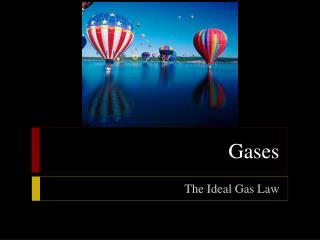Download PresentationGases

Loading in 2 Seconds...

# Gases - PowerPoint PPT Presentation

Gases. The Ideal Gas Law. The Ideal Gas Law. Objectives State the ideal gas law Using the ideal gas law, calculate pressure, volume, temperature, or amount of gas when the other three quantities are known. The Ideal Gas Law. Molar Volume of a GasI am the owner, or an agent authorized to act on behalf of the owner, of the copyrighted work described.
Download Presentation## Gases

An Image/Link below is provided (as is) to download presentation

Download Policy: Content on the Website is provided to you AS IS for your information and personal use and may not be sold / licensed / shared on other websites without getting consent from its author.While downloading, if for some reason you are not able to download a presentation, the publisher may have deleted the file from their server.

- - - - - - - - - - - - - - - - - - - - - - - - - - E N D - - - - - - - - - - - - - - - - - - - - - - - - - -
Presentation Transcript
1. Gases The Ideal Gas Law

2. The Ideal Gas Law • Objectives • State the ideal gas law • Using the ideal gas law, calculate pressure, volume, temperature, or amount of gas when the other three quantities are known

3. The Ideal Gas Law • Molar Volume of a Gas • According to Avogadro’s law, V = kn (where n = number of moles of a gas), one mole of any gas will occupy the same volume as one mole of any other gas at the same conditions, despite mass differences • STP is the standard temperature and pressure of a gas (273K and 1atm) • The volume occupied by one mole of gas at STP is known as the standard molar volume of a gas, which is 24.41410 L (rounded to 22.4 L)

4. The Ideal Gas Law • Molar Volume of a Gas • Knowing the volume of a gas, you can use the conversion factor 1 mol/22.4 L to find the moles (and therefore also mass) of a given volume of gas at STP Example: at STP, 5.00 L of gas x = 0.223 mol of gas • You can also use the molar volume of a gas to find the volume, at STP, of a known number of moles or a known mass of gas Example: at STP, 0.768 mol of gas x = 17.2 L of gas 1 mol 22.4 L 22.4 L 1 mol

5. Gas Stoichiometry • For gaseous reactants or products, the coefficients in chemical equations not only indicate molar amounts and mole ratios but also reveal volume ratios, assuming conditions remain the same. • Example: 2CO + O2 →2CO2 2 volumes 1 volume 2 volumes

6. The Ideal Gas Law • The Ideal Gas Law • You have learned about equations describing the relationships between two or three of the four variables—pressure, volume, temperature, and moles—needed to describe a gas sample at a time • All of the gas laws you have learned thus far can be combined into a single equation, the ideal gas law: the mathematical relationship among pressure, volume, temperature, and number of moles of a gas

7. The Ideal Gas Law • The Ideal Gas Law • It is stated as shown below, where R is a constant: PV = nRT • P = pressure • V = volume • n = number of moles of gas • T = temperature • R = constant

8. The Ideal Gas Law • The Ideal Gas Law Constant • In the equation representing the ideal gas law, the constant R is known as the ideal gas constant • Its value depends on the units chosen for pressure, volume, and temperature in the rest of the equation • Measured values of P, V, T, and n for a gas at near-ideal conditions can be used to calculate R:

9. The Ideal Gas Law • The Ideal Gas Law Constant • The calculated value of R is usually rounded to 0.0821 (L•atm)/(mol•K) • Use this value in ideal gas law calculations when the volume is in liters, the pressure is in atmospheres, and the temperature is in kelvins • The ideal gas law can be applied to determine the existing conditions of a gas sample when three of the four values, P, V, T, and n, are known • Be sure to match the units of the known quantities and the units of R

10. The Ideal Gas Law • Numerical Values of the Ideal Gas Law Constant

11. The Gas Laws • The Ideal Gas Law • Sample Problem • What is the pressure in atmospheres exerted by a 0.500 mol sample of nitrogen gas in a 10.0 L container at 298 K?

12. The Gas Laws • The Ideal Gas Law • Sample Problem Solution • Given: V of N2 = 10.0 L n of N2 = 0.500 mol • T of N2 = 298 K • Unknown: P of N2 in atm